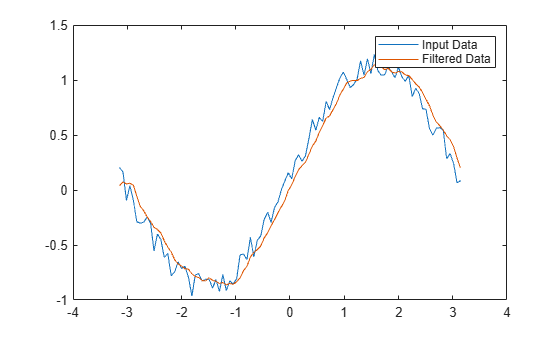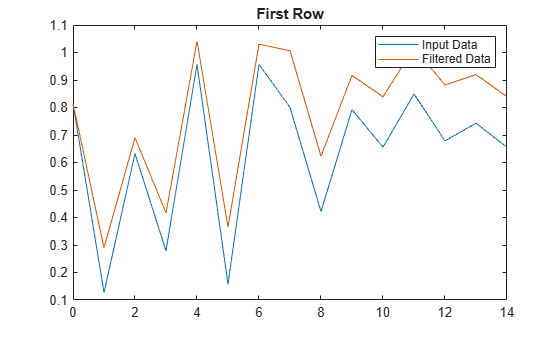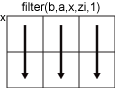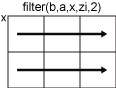# filter

1-D digital filter

## Syntax

``y = filter(b,a,x)``
``y = filter(b,a,x,zi)``
``y = filter(b,a,x,zi,dim)``
``````[y,zf] = filter(___)``````

## Description

example

````y = filter(b,a,x)` filters the input data `x` using a rational transfer function defined by the numerator and denominator coefficients `b` and `a`.If `a(1)` is not equal to `1`, then `filter` normalizes the filter coefficients by `a(1)`. Therefore, `a(1)` must be nonzero. If `x` is a vector, then `filter` returns the filtered data as a vector of the same size as `x`.If `x` is a matrix, then `filter` acts along the first dimension and returns the filtered data for each column.If `x` is a multidimensional array, then `filter` acts along the first array dimension whose size does not equal 1. ```

example

````y = filter(b,a,x,zi)` uses initial conditions `zi` for the filter delays. The length of `zi` must equal `max(length(a),length(b))-1`.```

example

````y = filter(b,a,x,zi,dim)` acts along dimension `dim`. For example, if `x` is a matrix, then `filter(b,a,x,zi,2)` returns the filtered data for each row.```

example

``````[y,zf] = filter(___)``` also returns the final conditions `zf` of the filter delays, using any of the previous syntaxes.```

## Examples

collapse all

A moving-average filter is a common method used for smoothing noisy data. This example uses the `filter` function to compute averages along a vector of data.

Create a 1-by-100 row vector of sinusoidal data that is corrupted by random noise.

```t = linspace(-pi,pi,100); rng default %initialize random number generator x = sin(t) + 0.25*rand(size(t));```

A moving-average filter slides a window of length $windowSize$ along the data, computing averages of the data contained in each window. The following difference equation defines a moving-average filter of a vector $x$:

`$y\left(n\right)=\frac{1}{windowSize}\left(x\left(n\right)+x\left(n-1\right)+...+x\left(n-\left(windowSize-1\right)\right)\right).$`

For a window size of 5, compute the numerator and denominator coefficients for the rational transfer function.

```windowSize = 5; b = (1/windowSize)*ones(1,windowSize); a = 1;```

Find the moving average of the data and plot it against the original data.

```y = filter(b,a,x); plot(t,x) hold on plot(t,y) legend('Input Data','Filtered Data')```This example filters a matrix of data with the following rational transfer function.

`$H\left(z\right)=\frac{b\left(1\right)}{a\left(1\right)+a\left(2\right){z}^{-1}}=\frac{1}{1-0.2{z}^{-1}}$`

Create a 2-by-15 matrix of random input data.

```rng default %initialize random number generator x = rand(2,15);```

Define the numerator and denominator coefficients for the rational transfer function.

```b = 1; a = [1 -0.2];```

Apply the transfer function along the second dimension of `x` and return the 1-D digital filter of each row. Plot the first row of original data against the filtered data.

```y = filter(b,a,x,[],2); t = 0:length(x)-1; %index vector plot(t,x(1,:)) hold on plot(t,y(1,:)) legend('Input Data','Filtered Data') title('First Row')```Plot the second row of input data against the filtered data.

```figure plot(t,x(2,:)) hold on plot(t,y(2,:)) legend('Input Data','Filtered Data') title('Second Row')```Use initial and final conditions for filter delays to filter data in sections, especially if memory limitations are a consideration.

Generate a large random data sequence and split it into two segments, `x1` and `x2`.

```x = randn(10000,1); x1 = x(1:5000); x2 = x(5001:end);```

The whole sequence, `x`, is the vertical concatenation of `x1` and `x2`.

Define the numerator and denominator coefficients for the rational transfer function,

`$H\left(z\right)=\frac{b\left(1\right)+b\left(2\right){z}^{-1}}{a\left(1\right)+a\left(2\right){z}^{-1}}=\frac{2+3{z}^{-1}}{1+0.2{z}^{-1}}.$`

```b = [2,3]; a = [1,0.2];```

Filter the subsequences `x1` and `x2` one at a time. Output the final conditions from filtering `x1` to store the internal status of the filter at the end of the first segment.

`[y1,zf] = filter(b,a,x1);`

Use the final conditions from filtering `x1` as initial conditions to filter the second segment, `x2`.

`y2 = filter(b,a,x2,zf);`

`y1` is the filtered data from `x1`, and `y2` is the filtered data from `x2`. The entire filtered sequence is the vertical concatenation of `y1` and `y2`.

Filter the entire sequence simultaneously for comparison.

```y = filter(b,a,x); isequal(y,[y1;y2])```
```ans = logical 1 ```

## Input Arguments

collapse all

Numerator coefficients of the rational transfer function, specified as a vector.

Data Types: `double` | `single` | `int8` | `int16` | `int32` | `int64` | `uint8` | `uint16` | `uint32` | `uint64` | `logical`
Complex Number Support: Yes

Denominator coefficients of the rational transfer function, specified as a vector.

Data Types: `double` | `single` | `int8` | `int16` | `int32` | `int64` | `uint8` | `uint16` | `uint32` | `uint64` | `logical`
Complex Number Support: Yes

Input data, specified as a vector, matrix, or multidimensional array.

Data Types: `double` | `single` | `int8` | `int16` | `int32` | `int64` | `uint8` | `uint16` | `uint32` | `uint64` | `logical`
Complex Number Support: Yes

Initial conditions for filter delays, specified as a vector, matrix, or multidimensional array.

• If `zi` is a vector, then its length must be `max(length(a),length(b))-1`.

• If `zi` is a matrix or multidimensional array, then the size of the leading dimension must be `max(length(a),length(b))-1`. The size of each remaining dimension must match the size of the corresponding dimension of `x`. For example, consider using `filter` along the second dimension (`dim = 2`) of a 3-by-4-by-5 array `x`. The array `zi` must have size [`max(length(a),length(b))-1`]-by-3-by-5.

The default value, specified by `[]`, initializes all filter delays to zero.

Data Types: `double` | `single` | `int8` | `int16` | `int32` | `int64` | `uint8` | `uint16` | `uint32` | `uint64` | `logical`
Complex Number Support: Yes

Dimension to operate along, specified as a positive integer scalar. If no value is specified, then the default is the first array dimension whose size does not equal 1.

Consider a two-dimensional input array, `x`.

• If `dim = 1`, then `filter(b,a,x,zi,1)`works along the rows of `x` and returns the filter applied to each column.• If `dim = 2`, then `filter(b,a,x,zi,2)` works along the columns of `x` and returns the filter applied to each row.If `dim` is greater than `ndims(x)`, then `filter` returns `x`.

Data Types: `double` | `single` | `int8` | `int16` | `int32` | `int64` | `uint8` | `uint16` | `uint32` | `uint64` | `logical`

## Output Arguments

collapse all

Filtered data, returned as a vector, matrix, or multidimensional array of the same size as the input data, `x`.

If `x` is of type `single`, then `filter` natively computes in single precision, and `y` is also of type `single`. Otherwise, `y` is returned as type `double`.

Data Types: `double` | `single`

Final conditions for filter delays, returned as a vector, matrix, or multidimensional array.

• If `x` is a vector, then `zf` is a column vector of length `max(length(a),length(b))-1`.

• If `x` is a matrix or multidimensional array, then `zf` is an array of column vectors of length `max(length(a),length(b))-1`, such that the number of columns in `zf` is equivalent to the number of columns in `x`. For example, consider using `filter` along the second dimension (`dim = 2`) of a 3-by-4-by-5 array `x`. The array `zf` has size [`max(length(a),length(b))-1`]-by-3-by-5.

Data Types: `double` | `single`

collapse all

### Rational Transfer Function

The input-output description of the `filter` operation on a vector in the Z-transform domain is a rational transfer function. A rational transfer function is of the form

`$Y\left(z\right)=\frac{b\left(1\right)+b\left(2\right){z}^{-1}+...+b\left({n}_{b}+1\right){z}^{-{n}_{b}}}{1+a\left(2\right){z}^{-1}+...+a\left({n}_{a}+1\right){z}^{-{n}_{a}}}X\left(z\right),$`

which handles both FIR and IIR filters . na is the feedback filter order, and nb is the feedforward filter order. Due to normalization, assume a(1) = 1.

You also can express the rational transfer function as the difference equation

`$\begin{array}{c}a\left(1\right)y\left(n\right)=b\left(1\right)x\left(n\right)+b\left(2\right)x\left(n-1\right)+...+b\left({n}_{b}+1\right)x\left(n-{n}_{b}\right)\\ -a\left(2\right)y\left(n-1\right)-...-a\left({n}_{a}+1\right)y\left(n-{n}_{a}\right).\end{array}$`

Furthermore, you can represent the rational transfer function using its direct-form II transposed implementation, as in the following diagram. Here, na = nb.The operation of `filter` at sample m is given by the time-domain difference equations

## Tips

• If you have Signal Processing Toolbox™, use `y = filter(d,x)` to filter an input signal `x` with a `digitalFilter` object `d`. To generate `d` based on frequency-response specifications, use `designfilt`.

• If you have DSP System Toolbox™, use `y = filter(dObj,x)` to filter an input signal `x` with a `dfilt` object `dObj`.

• To use the `filter` function with the `b` coefficients from an FIR filter, use ```y = filter(b,1,x)```.

• See Digital Filtering (Signal Processing Toolbox) for more on filtering functions.

 Oppenheim, Alan V., Ronald W. Schafer, and John R. Buck. Discrete-Time Signal Processing. Upper Saddle River, NJ: Prentice-Hall, 1999.